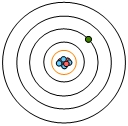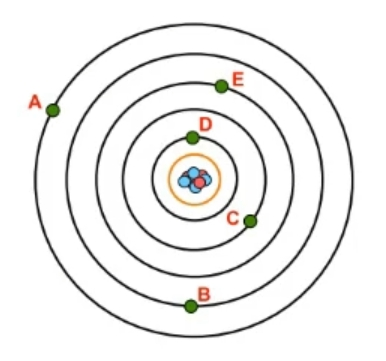## General Chemistry

Learn the toughest concepts covered in Chemistry with step-by-step video tutorials and practice problems by world-class tutors

9. Quantum Mechanics

# Quantum Numbers: Principal Quantum Number

The Principal Quantum Number gives both the size and energy of an electron shell.

Principal Quantum Number
1
concept

## Quantum Numbers: Principal Quantum Number2m
Play a video:
the first quantum number of interest is the principal quantum number. It uses the variable end and the principal quantum number of deals with the signs off the shell and the size of a shell is equal to the energy level of that show. What we need to realize here, as the value of for an increases than both the size and energy level of an atomic orbital will also increase. Now we're going to say here that the energy levels or shell numbers often Adam can be tied to the periods or rose of the periodic table. So here we take a look at an atom. Here we have our nucleus in the middle. We have Here are first shell so n equals one all the way to our seventh shell here, which is an equal seven. These shell numbers are connected to the periods or rows of the periodic table. So shall one is connected to period one. And as you can see, we want all the way up to to shell seven and looking at the periodic table. We have periods. One, 27 Now here's the thing. The periodic table. If you've watched my videos on it. We know that the periodic table is not a static thing, meaning that it's not going to stay in this form forever. In fact, period seven ah, lot of those elements in Period seven were only discovered within the past century. That's because, as we explore new places on Earth as we explore the universe, as our technology becomes more advanced will discover new elements will create new elements. So theoretically, the number of rows in the periodic table is infinite. Right now we have seven rows, but it could increase to eight rows 10 rolls in 1000 years. So just realize here that the number of rows for the periodic table doesn't stop it. Seven. It's only seven now because of where we are technologically. In the future there's gonna be more than seven rows, so we're gonna find atoms that go beyond seven. This ties into the limitation of the principal quantum number. We're going to say here that the principal quantum number n must be an integer so a whole number, and since it's connected to the periods of the periodic table, it has to start off at one at the lowest as its lowest possible value. And we just said to the number of rows is not static. It increases over time. So an theoretically can be any whole number from one to infinity. So just realized on an exam or quiz you C N equals 22. That's possible. We just haven't gotten there yet, so just remember the principal quantum numbers connected to the size and energy off a shell, and it affect the size and energy of the orbital's.
2
example

## Quantum Numbers: Principal Quantum Number Example 156s
Play a video:
here in this example question. It says which of the following is a possible value for a principal quantum number? Shell. So here we have an equals negative. Three negative 40 11 and negative seven. Remember, we said that your principal quantum number N is connected to the periods or rows of the periodic table. The smallest period value we can have a row is one. And we said that the periodic table is dynamic. It's always increasing. Right now we have seven rows, but in the future, we're gonna we're gonna have 89 10 rows. It can continue onward. So here, theoretically and equals any value from one to infinity. That means all these negative values would not work and and cannot equal zero has to start off as one as a possible value. So the answer here would have to be option D theoretically and can equal 11
3
Problem

What is the value of n for the electron based on the image of the atom provided?4
Problem

Which electron possesses the lowest possible energy from the image provided?